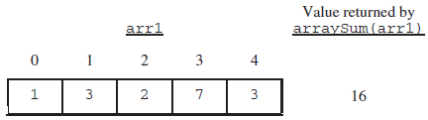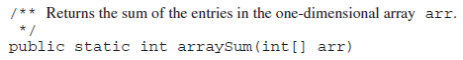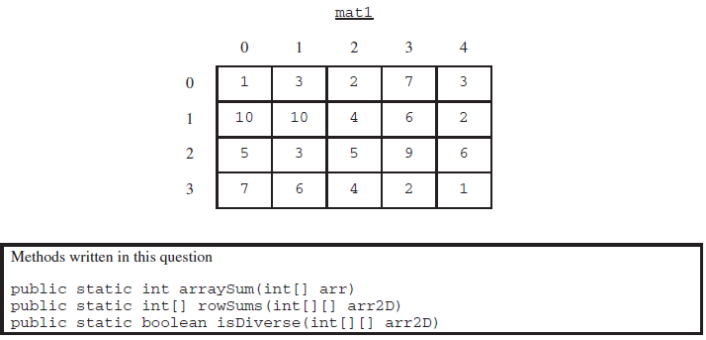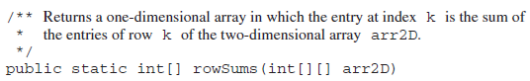# 2015 AP Computer Science A 计算机科学A真题系列之Released Exam简答题免费下载

### 真题与答案下载### 2015 AP 计算机科学A公开卷简答题部分免费下载

#### 部分真题预览：

1）This question involves reasoning about one-dimensional and two-dimensional arrays of integers. You will write three static methods, all of which are in a single enclosing class, named DiverseArray (not shown). The first method returns the sum of the values of a one-dimensional array; the second method returns an array that represents the sums of the rows of a two-dimensional array; and the third method analyzes row sums.

1. Write a static method arra Sum that calculates and returns the sum of the entries in a specified one-dimensional array. The following example shows an arr1 and the value returned by a call to arraySum.Complete method arraySum below.2. Write a static method rowSums that calculates the sums of each of the rows in a given two-dimensional array and returns these sums in a one-dimensional array. The method has one parameter, a two-dimensional array arr2D of int values. The array is in row-major order: arr2D [r] [c] is the entry at row r and column c. The method returns a one-dimensional array with one entry for each row of arr2D such that each entry is the sum of the corresponding row in arr2D . As a reminder, each row of a two-dimensional array is a one-dimensional array.
For example, if mat1 is the array represented by the following table, the call rowSums (mat1) returns the array {16,32,28,20}Assume that arraySum works as specified, regardless of what you wrote in part (a). You must use arraySum appropriately to receive full credit.
Complete method rowSums below.### 2015 AP Computer Science A 计算机科学公开卷FRQ简答题完整版答案免费下载